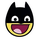# Introduction : Relative Strength Index (RSI)

Education
NSE:RCOM   RELIANCE COMM LTD370 views
Relative Strength Index is a so called momentum indicator that is very popular to use in technical analysis of financial instruments. Here’s a simple walkthrough of RSI and how to calculate.

The RSI was developed by J. Welles Wilder. The default setting for RSI is 14 Period or Candles, Which means that RSI evaluates past 14 candles.
The RSI Compares the average gain of up candles and average loss of down candles for past 14 candles.

The very first calculations for average gain and average loss is..
First Average Gain = Sum of Gains over the past 14 periods / 14.
First Average Loss = Sum of Losses over the past 14 periods / 14

The second, and subsequent, calculations are based on the prior averages and the current gain loss:
Average Gain = ((previous Average Gain) x 13 + current Gain) / 14.
Average Loss = ((previous Average Loss) x 13 + current Loss) / 14.

I Hope I kept it as Simple as I Can :) ( On Charts )
Do Let me know if I missed anything on RSI Introduction.

Thank you,
EEz

NEXT : Will Discuss Ways of using RSI Effectively.